# Data Analysis - Instakart¶

Before carrying out MBA on the given dataset, we can study the given dataset, its attributes and summarize the dataset for a better understanding.

In :
#imports
import pandas as pd #Python data analysis library
import numpy as np #Python scientific computing
import matplotlib.pyplot as plt #For plotting
import matplotlib.mlab as mlab
import seaborn as sns #Python visualization library
from scipy.optimize import curve_fit
from IPython.display import display, HTML

#Plots inline
%matplotlib inline


Citation - “The Instacart Online Grocery Shopping Dataset 2017”, Accessed from https://www.instacart.com/datasets/grocery-shopping-2017"

In :
#import dataset

In :
orderDf.shape

Out:
(3421083, 7)

There are 3421083 orders, roughly 35 lakh orders

In :
productDf.shape

Out:
(49688, 4)

There are 49688 products, roughly 50000 products

In :
#get distribution of number of orders per customer
sns.set_style('whitegrid')
customerNumOrderFrame = orderDf.groupby("user_id",as_index = False)["order_number"].max()
num_bins = 10
n, bins, patches = plt.hist(customerNumOrderFrame["order_number"] , num_bins, normed=1, color='blue', alpha=0.5)
mu = customerNumOrderFrame["order_number"].mean()
sigma = customerNumOrderFrame["order_number"].std()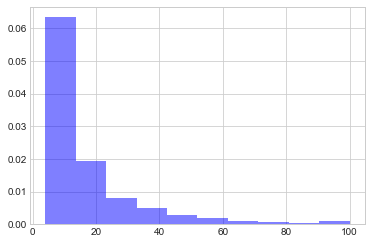Looking at the histogram it seems to be skewed and appropriate distribution will be an exponential function.

In :
n, bins, patches = plt.hist(customerNumOrderFrame["order_number"] , num_bins, normed=1, facecolor='yellow', alpha=0.5)

bins = np.delete(bins,10) #to make dimensions of x & y axis values equal
bins = bins+5 #to ge the central value of each bar

def exponenial_func(x, a, b, c):
return a*np.exp(-b*x)+c

popt, pcov = curve_fit(exponenial_func, bins, n, p0=(1, 1e-6, 1))

xx = np.linspace(8, 100, 30)
yy = exponenial_func(xx, *popt)

plt.plot(xx, yy ,'r--')
plt.xlabel("No. of Orders")
plt.ylabel("Count")
plt.title("Number of Orders per Customer Distribution")

Out:
Text(0.5,1,u'Number of Orders per Customer Distribution')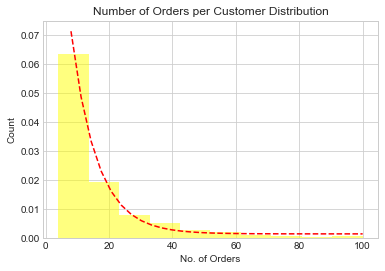Now to find the top frequently purchased products we will merge the prior and train dataset to get the complete order dataset. We will use append() for doing so.

In :
priorDf = pd.read_csv("../data/raw/order_products__prior.csv")
trainDf = trainDf.append(priorDf,ignore_index = True)
#Now a product count data frame can be created by counting the order_id for each product_id
productCountDf = trainDf.groupby("product_id",as_index = False)["order_id"].count()

In :
productCountDf.shape

Out:
(49685, 2)
In :
#Top 20 most frequently purchased products
topLev = 20

#Here order_id is the count so we need to sort the data frame w.r.t order_id
productCountDf = productCountDf.sort_values("order_id",ascending = False)

topProdFrame = productCountDf.iloc[0:topLev,:]
topProdFrame = topProdFrame.merge(productDf,on = "product_id")

display(topProdFrame.loc[:,["product_name"]])

product_name
0 Banana
1 Bag of Organic Bananas
2 Organic Strawberries
3 Organic Baby Spinach
6 Large Lemon
7 Strawberries
8 Limes
9 Organic Whole Milk
10 Organic Raspberries
11 Organic Yellow Onion
12 Organic Garlic
13 Organic Zucchini
14 Organic Blueberries
15 Cucumber Kirby
16 Organic Fuji Apple
17 Organic Lemon
18 Organic Grape Tomatoes
19 Apple Honeycrisp Organic

A density plot for product count can give an idea about to what extend we can perform smoothing of the dataset

In :
#Calculate the density
productCountDf["density"] = (productCountDf["order_id"]/np.sum(productCountDf["order_id"]))
#Calculate the rank
productCountDf["rank"] = range(productCountDf.shape)
plt.plot(productCountDf["rank"],productCountDf["density"])
plt.title("Density Plot for product counts")
plt.xlabel("Rank")
plt.ylabel("Density")

Out:
Text(0,0.5,u'Density')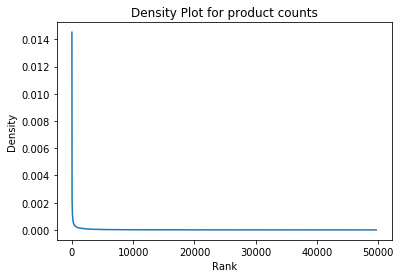It is hard to interpret much from this graph due to wide-ranging ranks (0-50000), So we can go for logarthmic scale for better visualization

In :
#Calculate log(Rank) also we add 1 to avoid log(0)
productCountDf["logRank"] = np.log(productCountDf["rank"] + 1)
plt.title("Density Plot for product counts")
plt.xlabel("$\log(Rank)$")
plt.ylabel("Density")
plt.plot(productCountDf["logRank"],productCountDf["density"])

Out:
[<matplotlib.lines.Line2D at 0x10994b310>]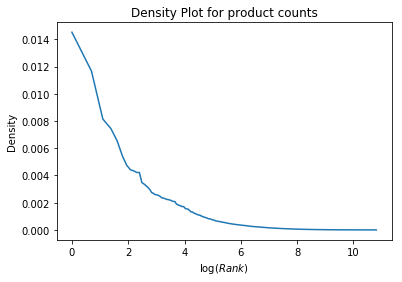We can see a very steep distribuiton and we can perform smoothening on the sparse distribuition area. e^6 = 403 products define most of the distribution. Products lying under (e^6, e^12) range are not significant since their respective density is very less.

Days of Orders in a week

In :
grouped = orderDf.groupby("order_id")["order_dow"].aggregate("sum").reset_index()
grouped = grouped.order_dow.value_counts()

sns.barplot(grouped.index, grouped.values)
plt.ylabel('Number of orders', fontsize=13)
plt.xlabel('Days of order in a week', fontsize=13)
plt.show()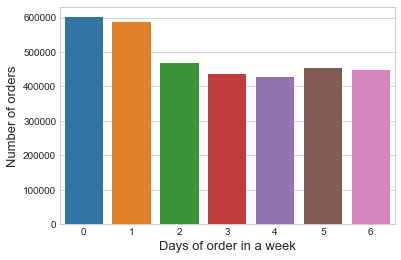Number of unique customers in the whole dataset-

In :
len(set(orderDf.user_id))

Out:
206209
In [ ]: